# RD Sharma Solutions Class 9 Maths Chapter 21 Surface Area And Volume Of Sphere

## RD Sharma Solutions Class 9 Maths Chapter 21 – Free PDF Download

RD Sharma Solutions for Class 9 Maths Chapter 21 Surface Area And Volume of a Sphere is given here which consists of questions and answers related to Spheres. These questions are really helpful for the preparation of board exams as well as other competitive exams. Here all solutions to questions in RD Sharma Solutions for Class 9 are given in a detailed and step by step format to help the students understand the concepts and clear all their doubts easily.

Students can find several exercises of Class 9 Maths Chapter 21 – Surface Area and Volume of Sphere. Click on the link below to download all the RD Sharma Solutions for in-depth learning of concepts. In this chapter, students will learn to find the surface area and volume of the sphere. Let us have a brief look at what is a sphere, In three-dimensional space, these are a set of points at an equal distance ‘r’ from a given point. The shape resembles a ball.

## Download PDF of RD Sharma Solutions for Class 9 Maths Chapter 21 Surface Area and Volume of A Sphere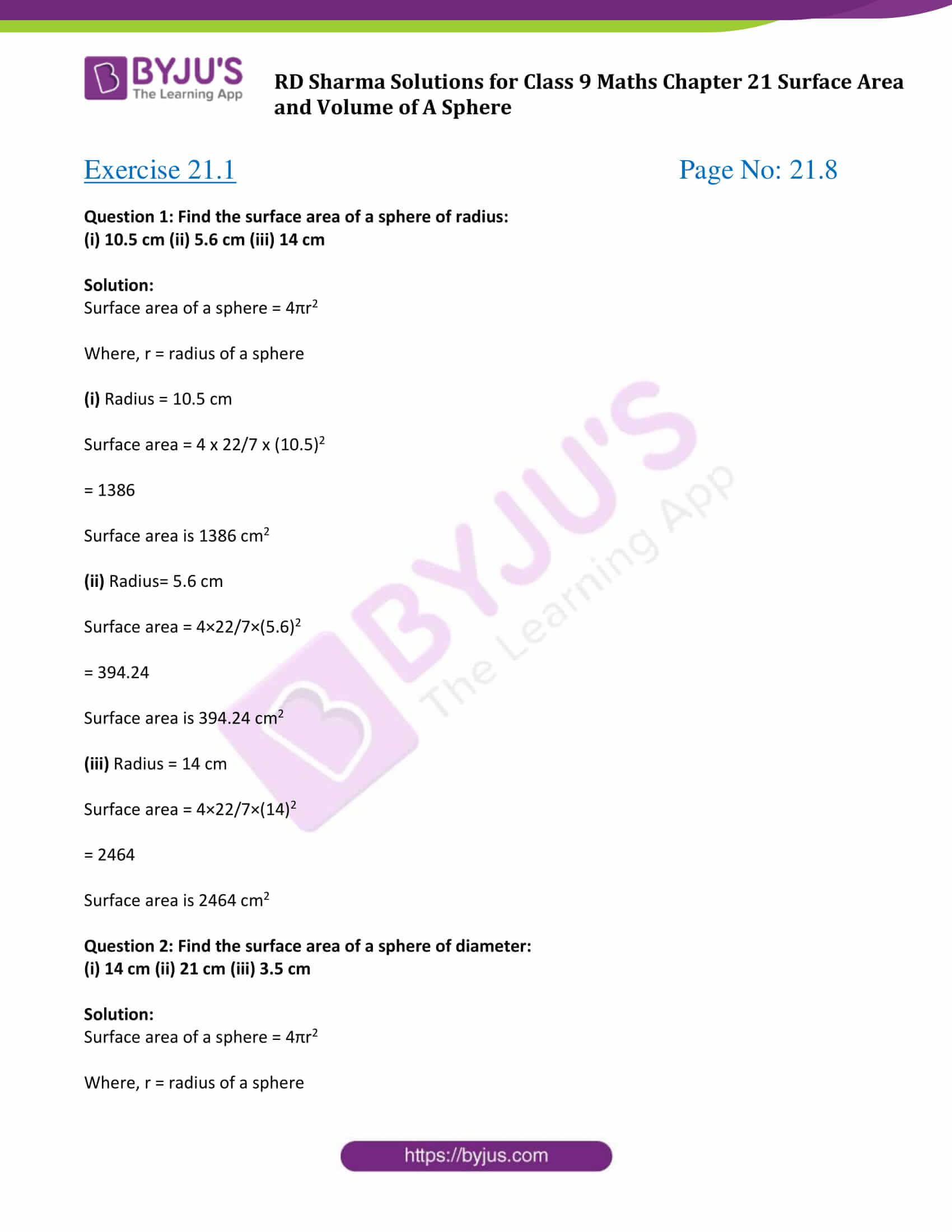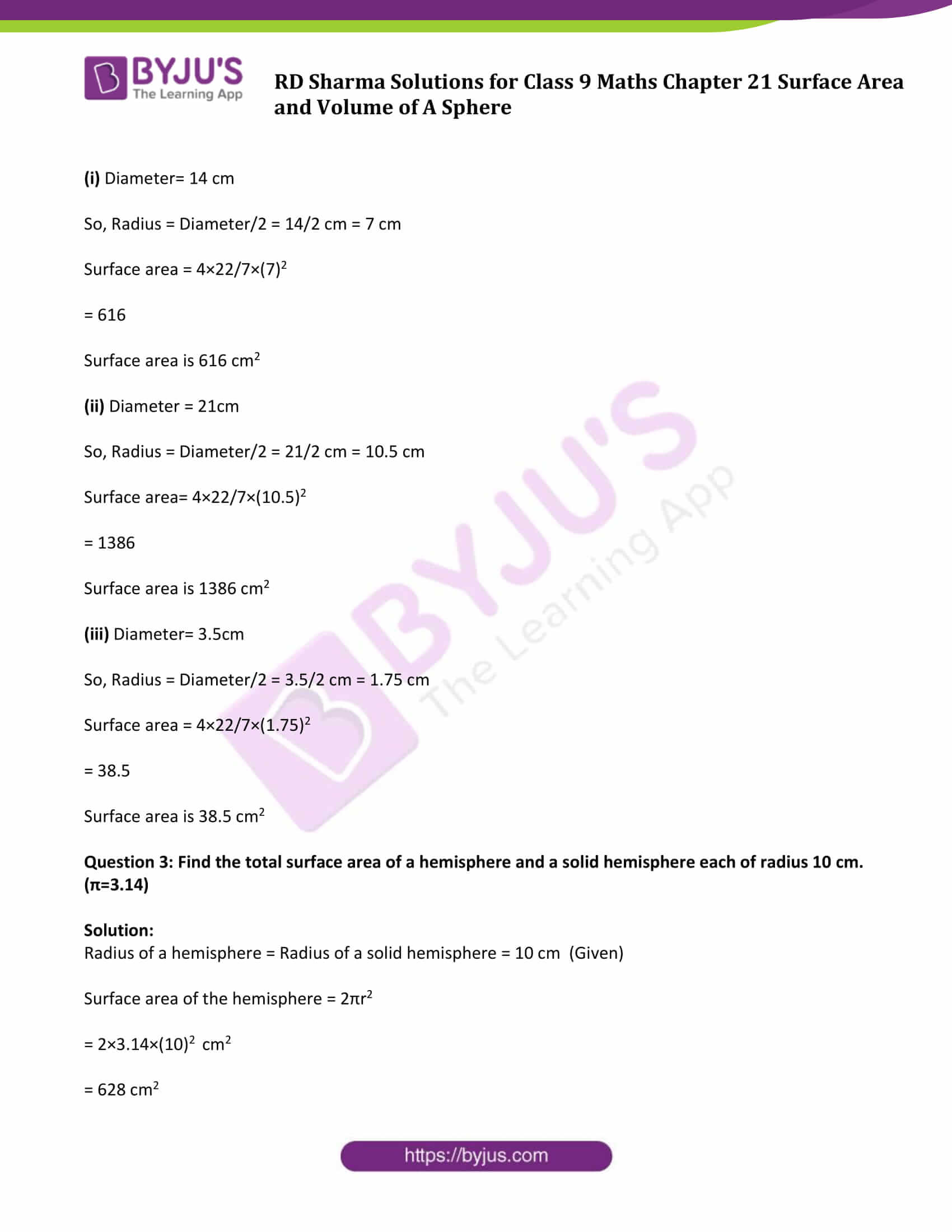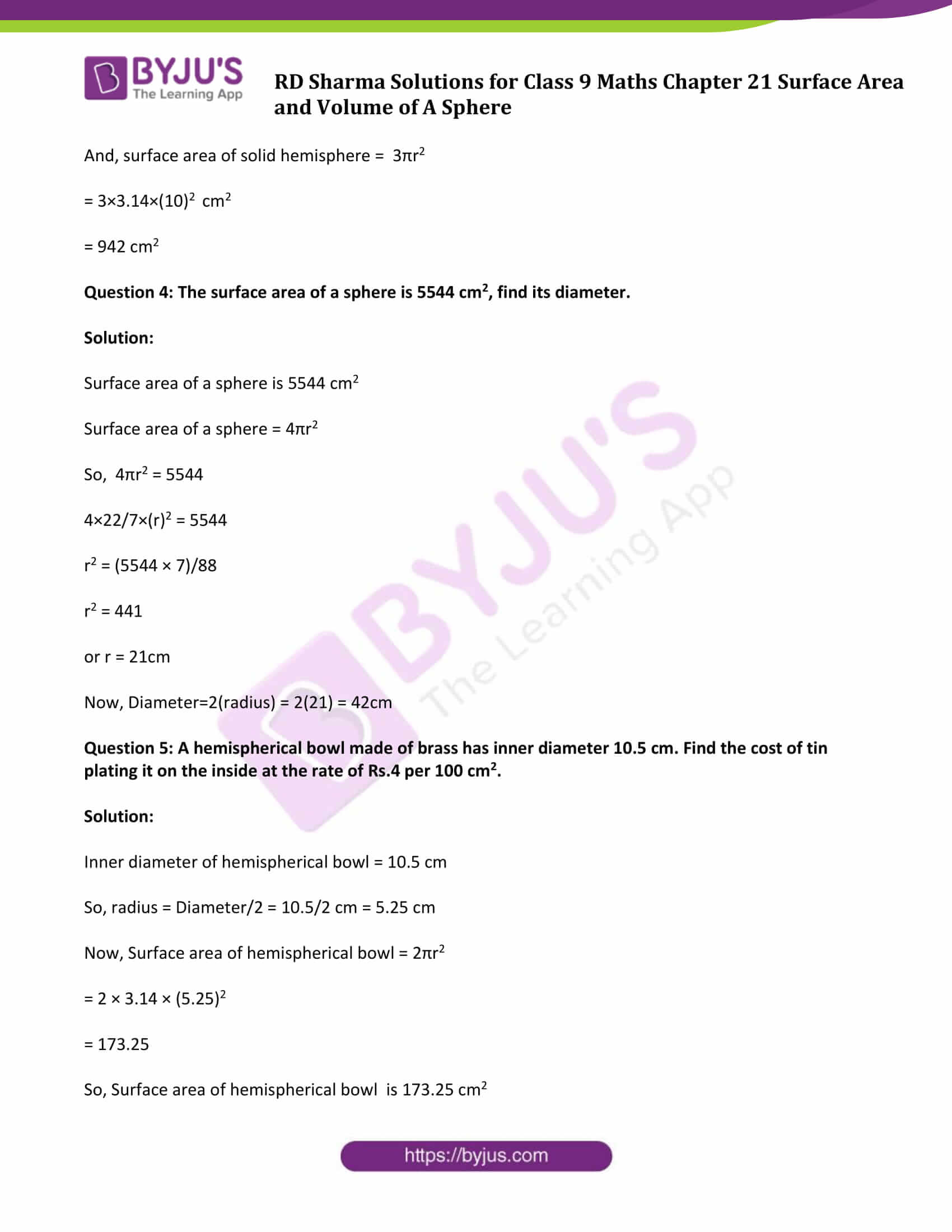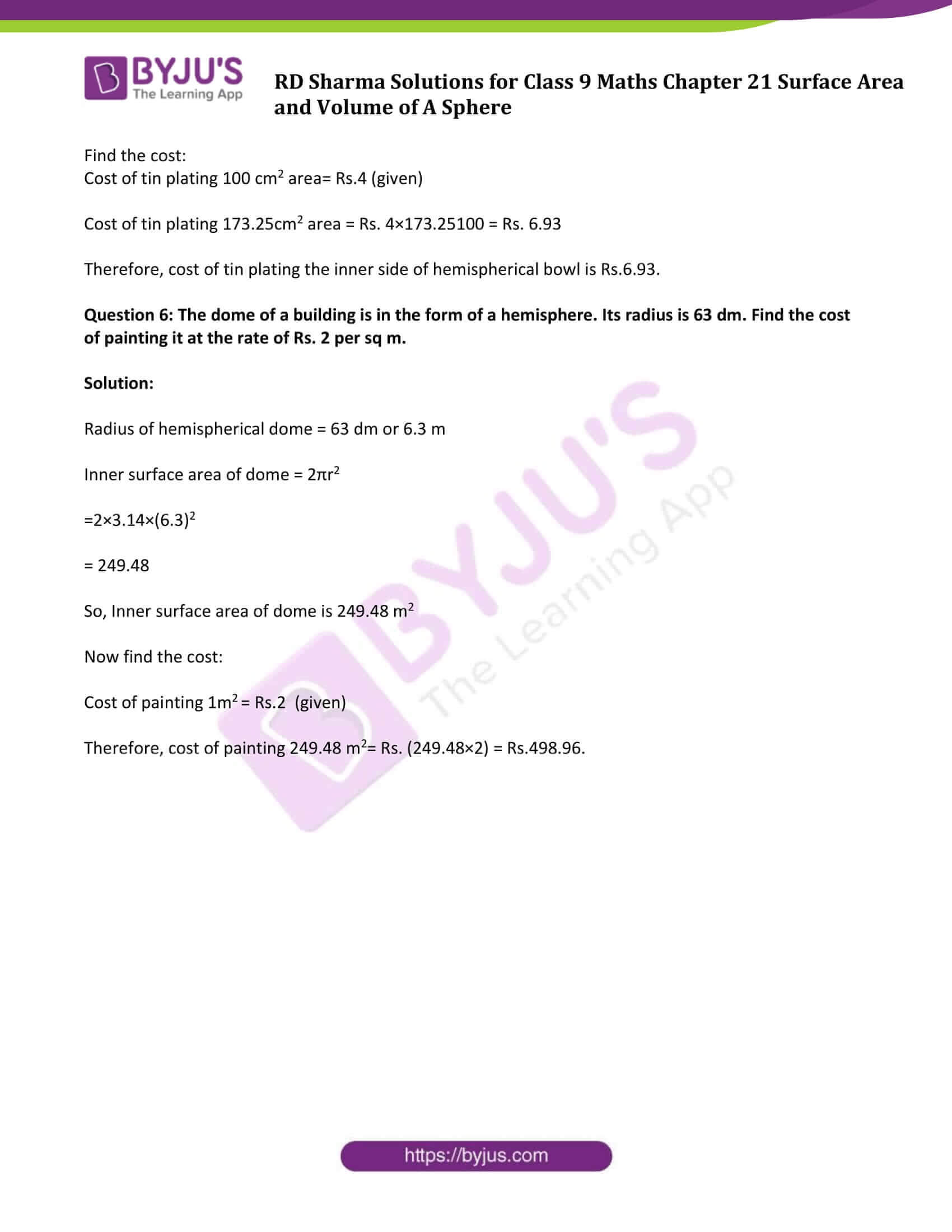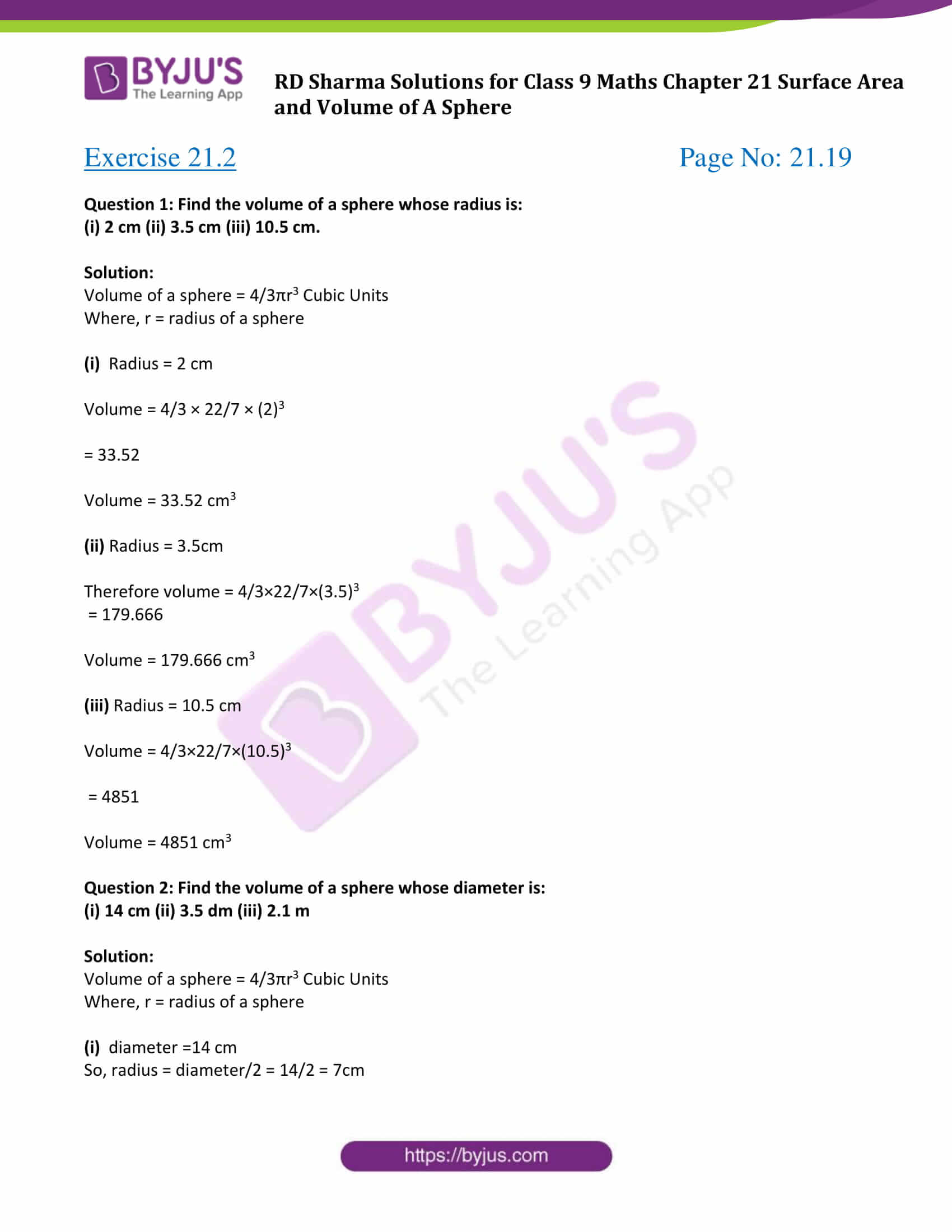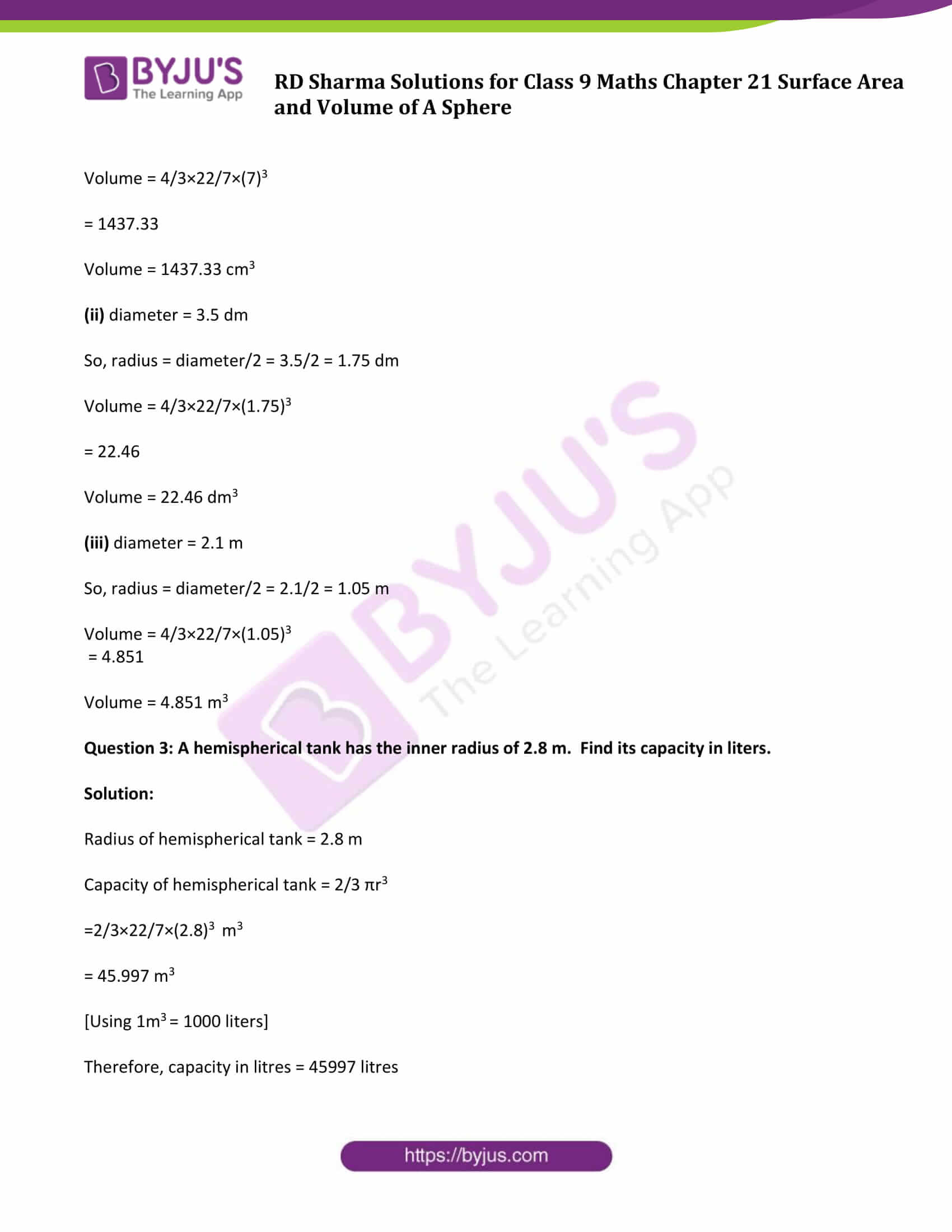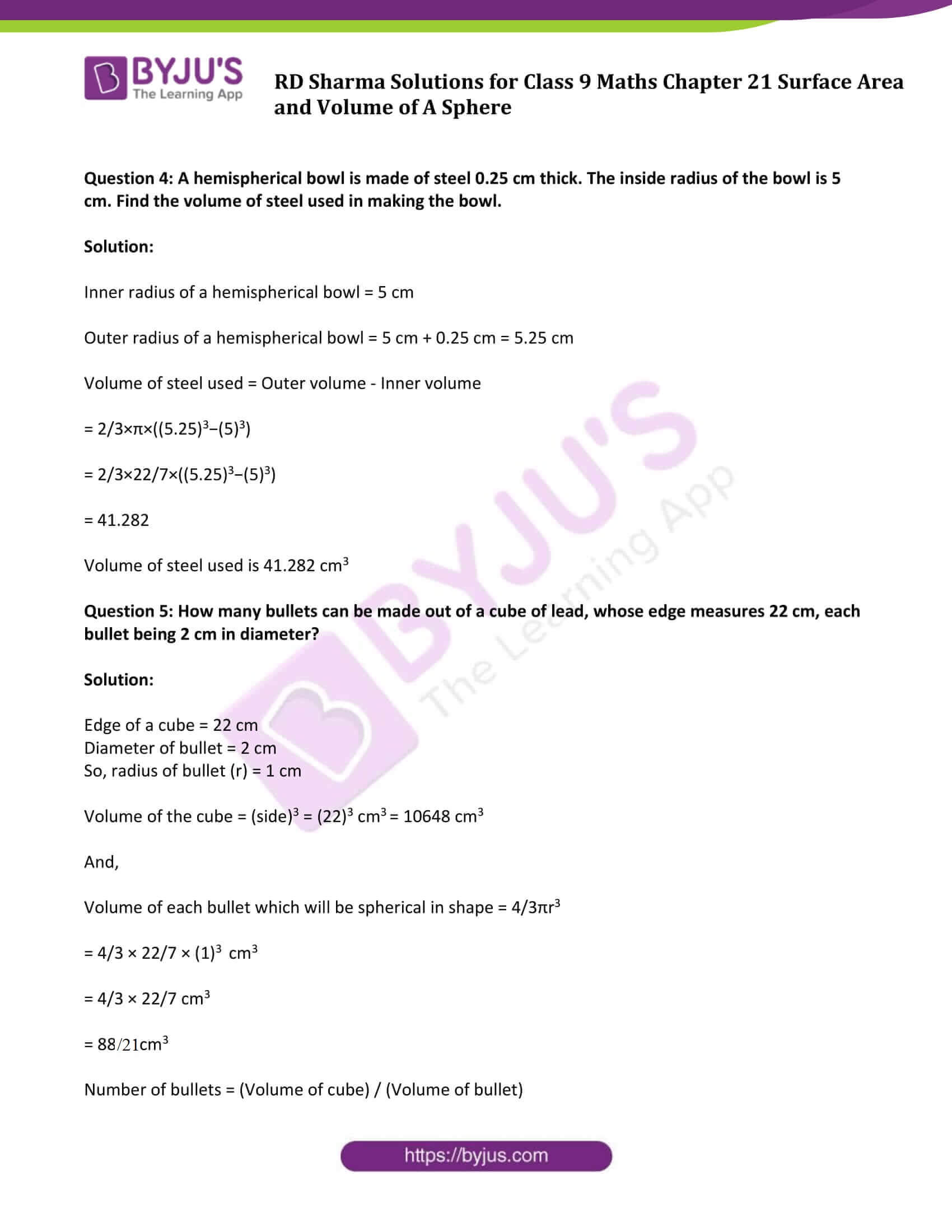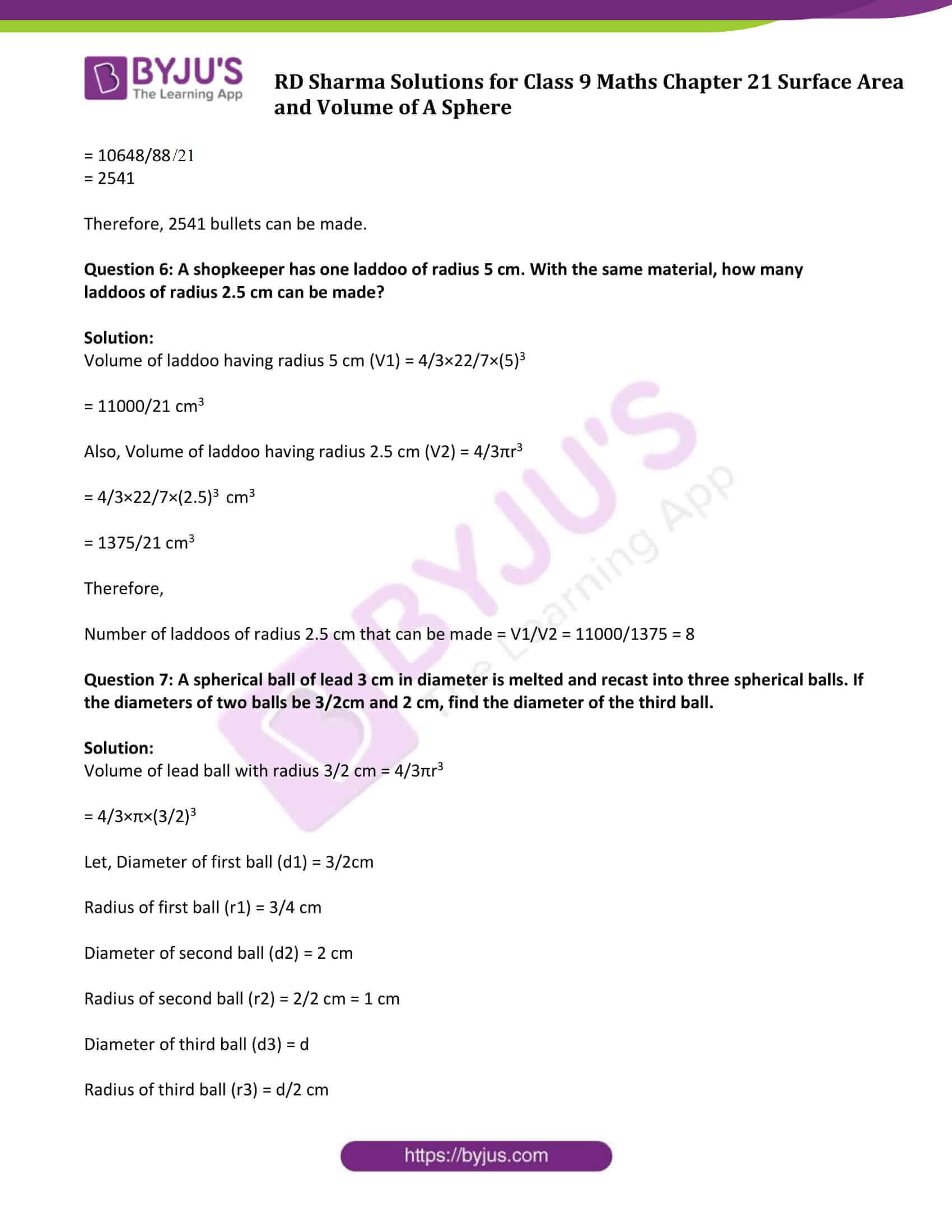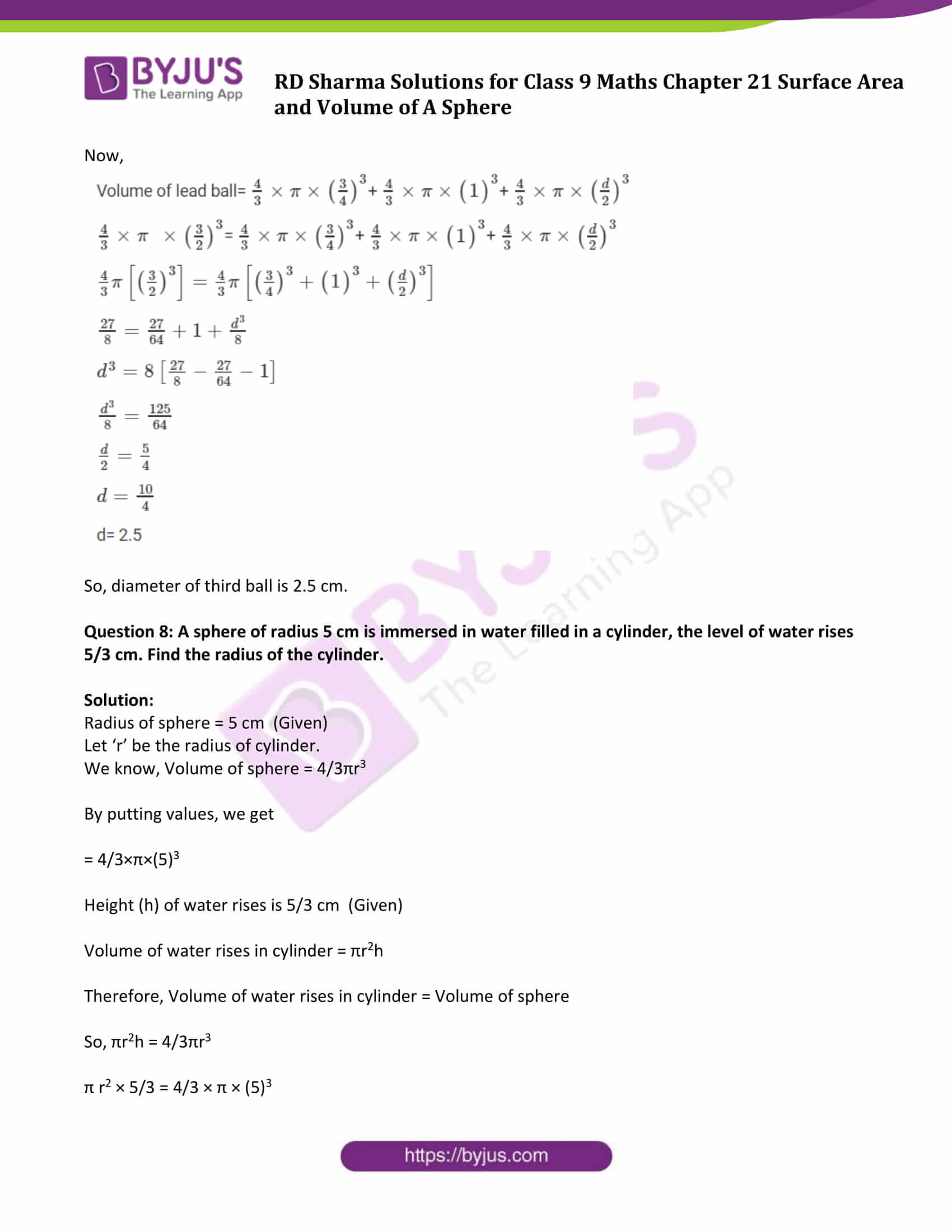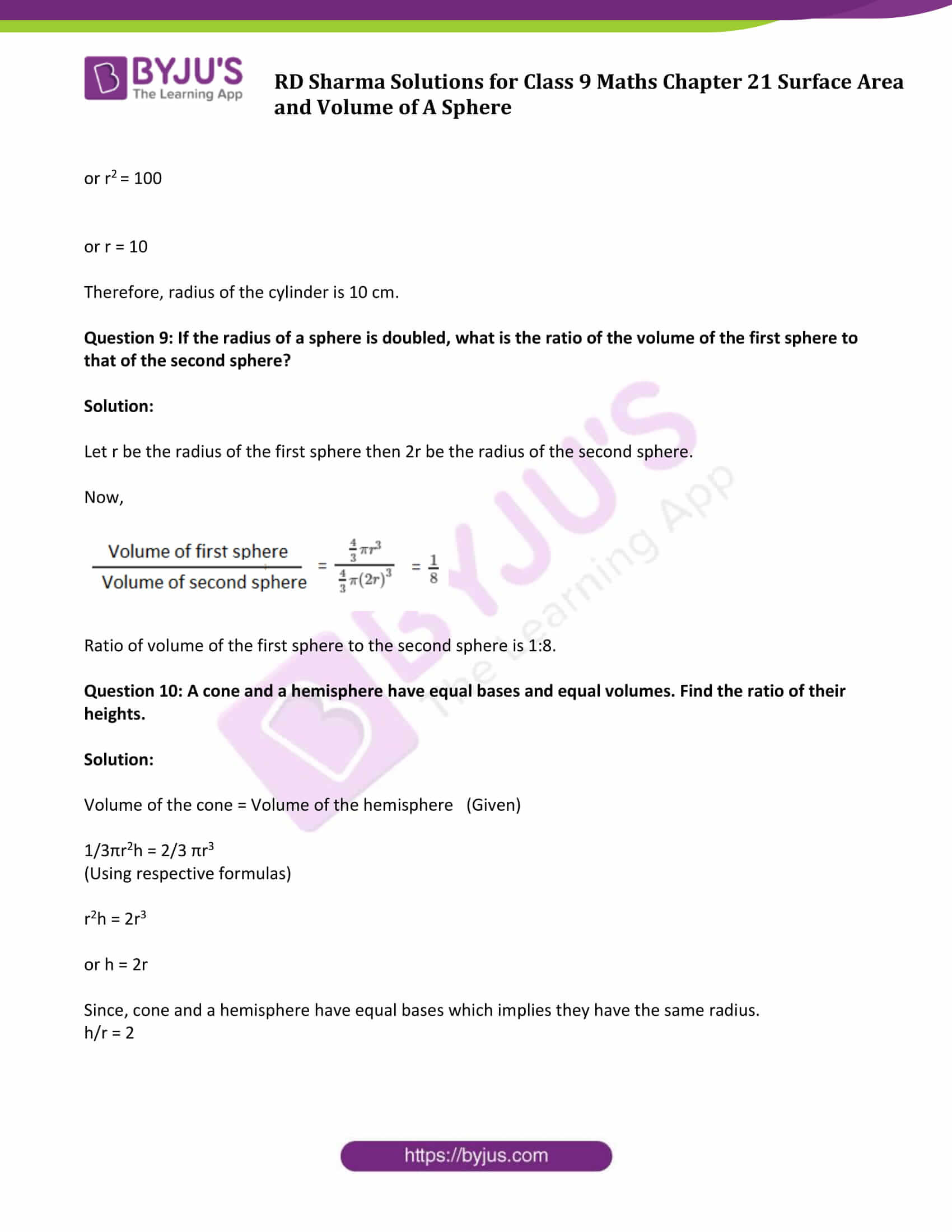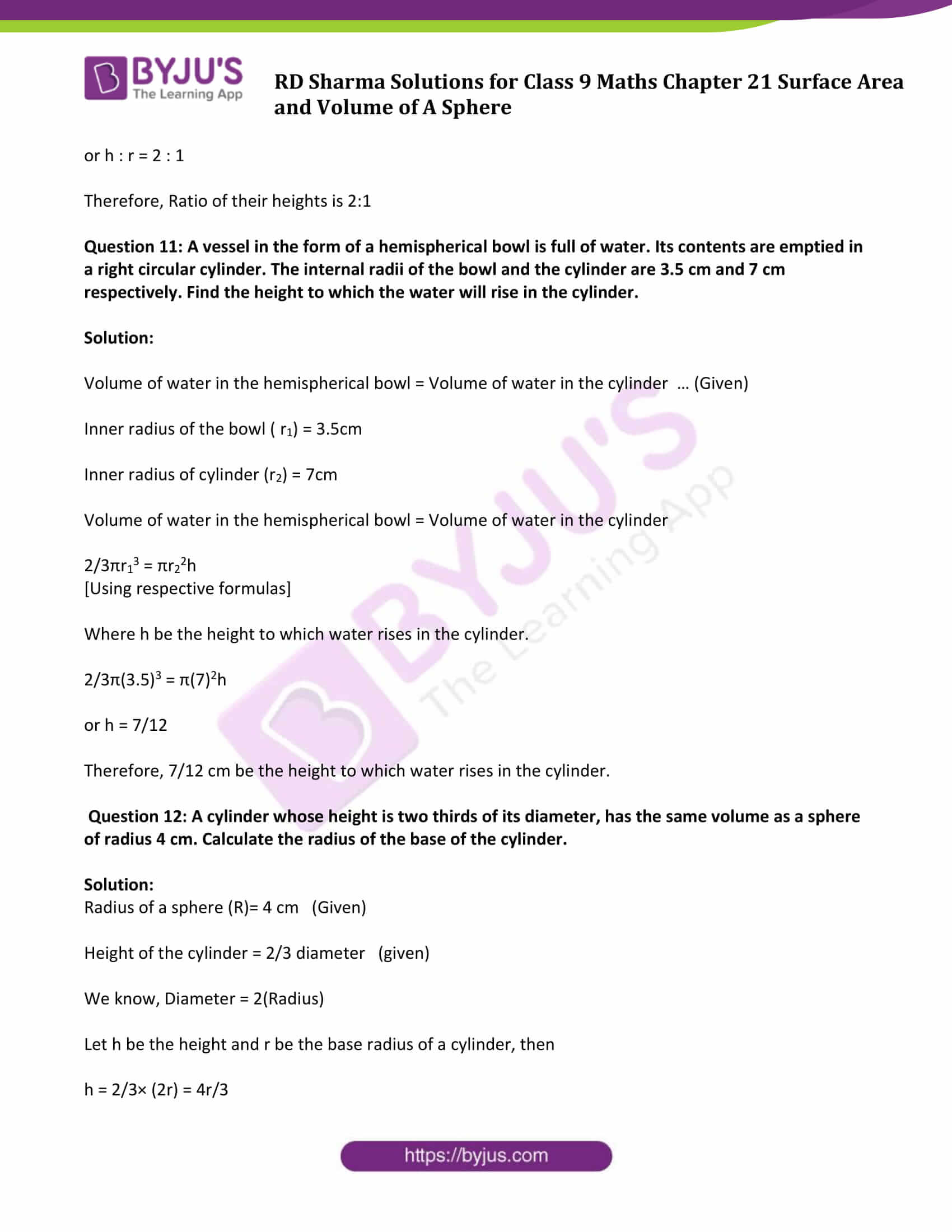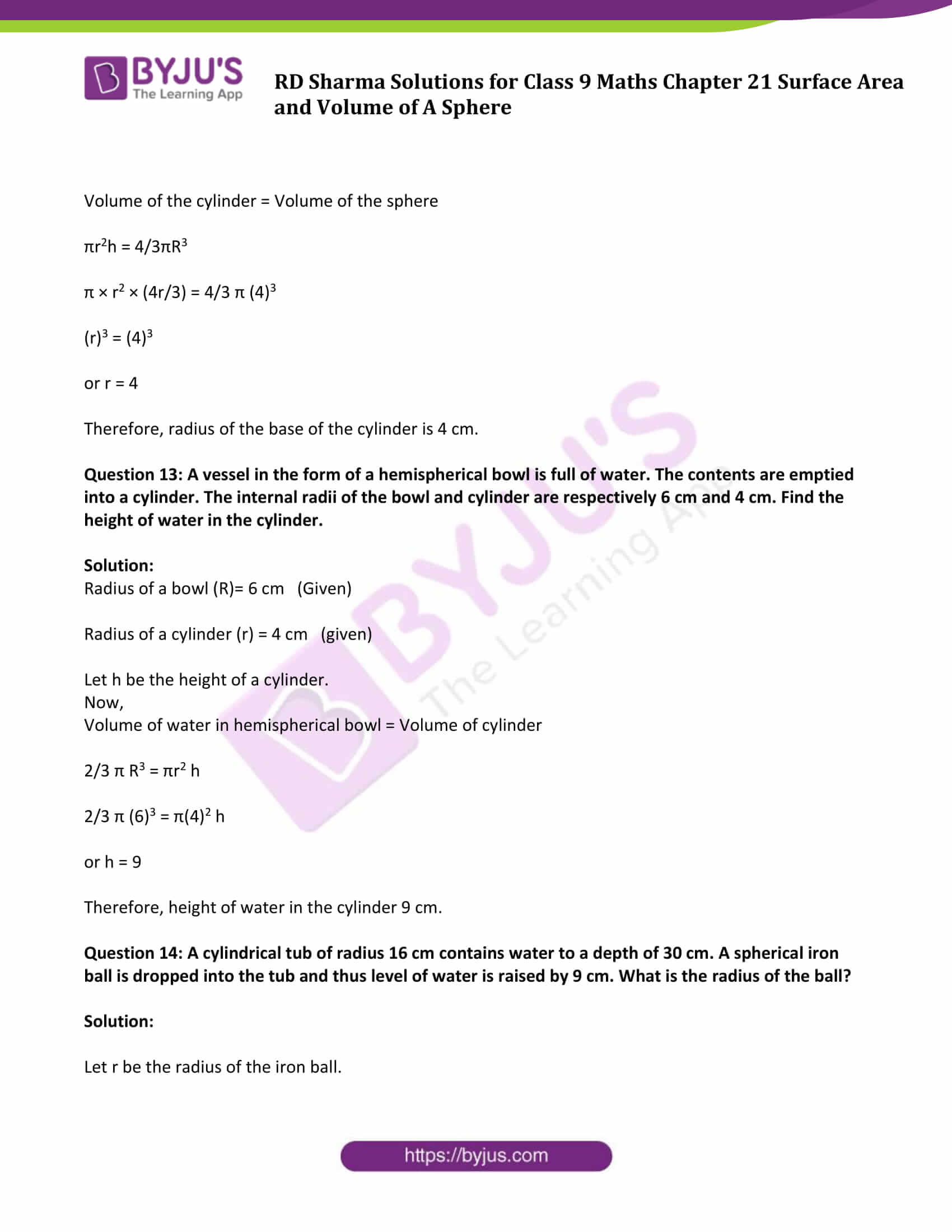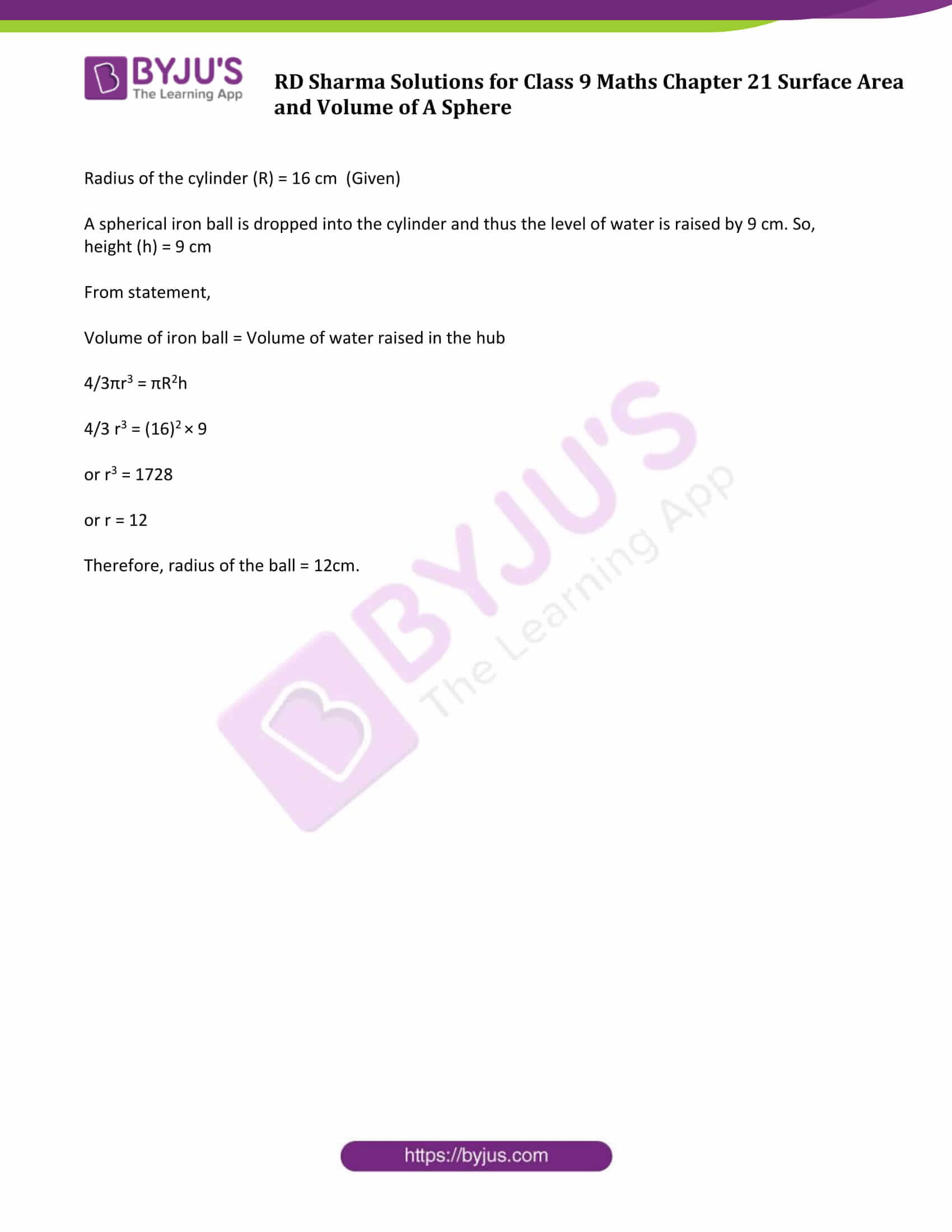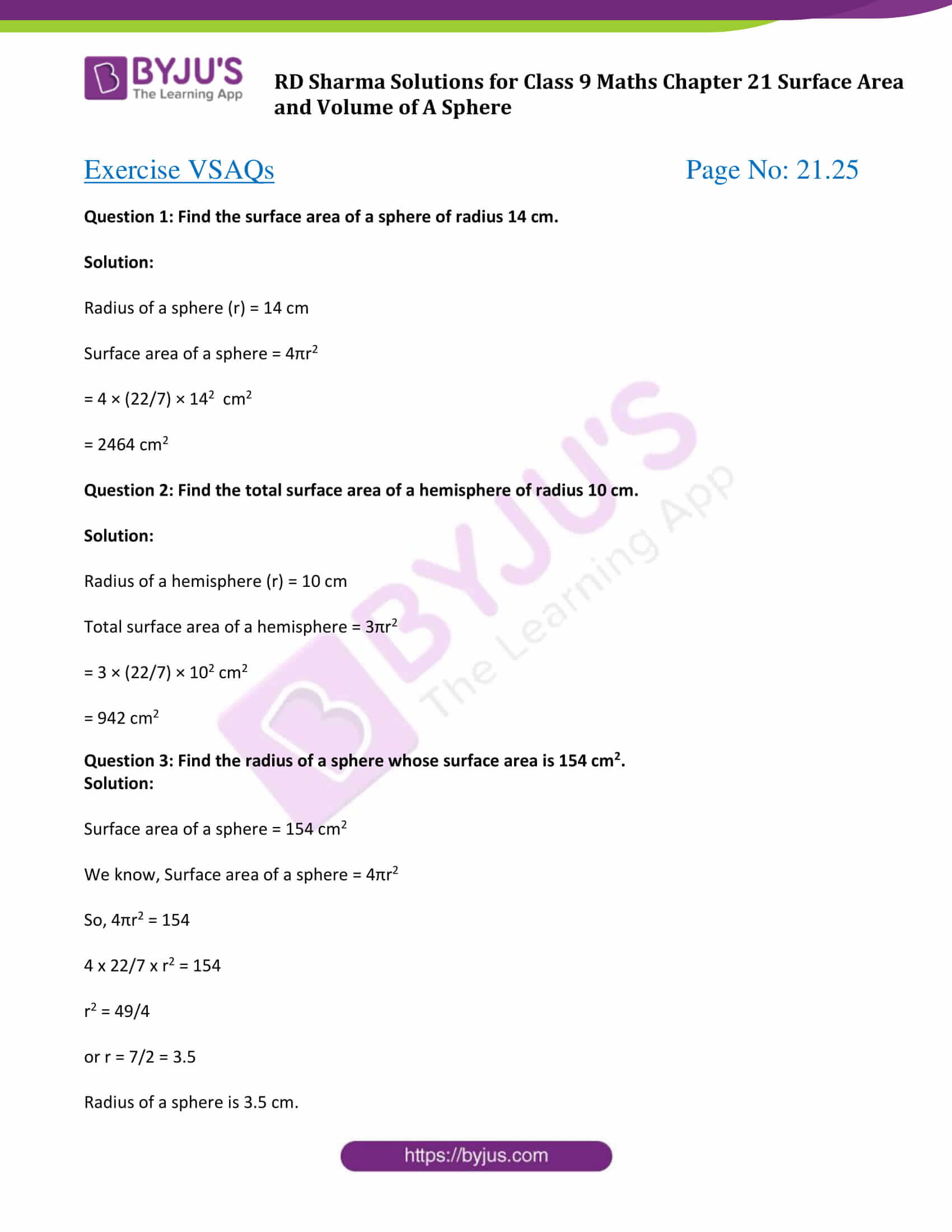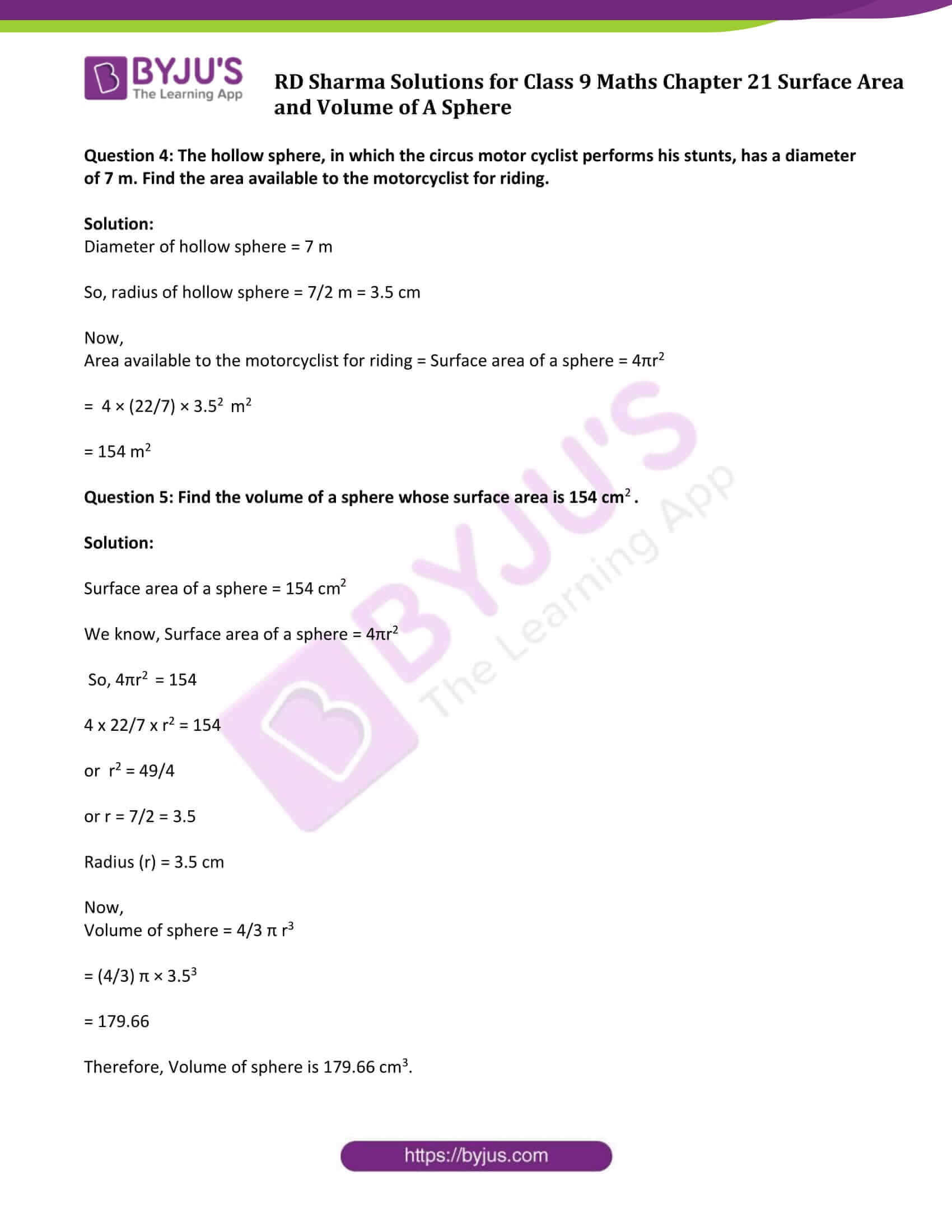### Exercise 21.1 Page No: 21.8

Question 1: Find the surface area of a sphere of radius:

(i) 10.5 cm (ii) 5.6 cm (iii) 14 cm

Solution:

Surface area of a sphere = 4πr2

Where, r = radius of a sphere

Surface area = 4 x 22/7 x (10.5)2

= 1386

Surface area is 1386 cm2

Surface area = 4×22/7×(5.6)2

= 394.24

Surface area is 394.24 cm2

Surface area = 4×22/7×(14)2

= 2464

Surface area is 2464 cm2

Question 2: Find the surface area of a sphere of diameter:

(i) 14 cm (ii) 21 cm (iii) 3.5 cm

Solution:

Surface area of a sphere = 4πr2

Where, r = radius of a sphere

(i) Diameter= 14 cm

So, Radius = Diameter/2 = 14/2 cm = 7 cm

Surface area = 4×22/7×(7)2

= 616

Surface area is 616 cm2

(ii) Diameter = 21cm

So, Radius = Diameter/2 = 21/2 cm = 10.5 cm

Surface area= 4×22/7×(10.5)2

= 1386

Surface area is 1386 cm2

(iii) Diameter= 3.5cm

So, Radius = Diameter/2 = 3.5/2 cm = 1.75 cm

Surface area = 4×22/7×(1.75)2

= 38.5

Surface area is 38.5 cm2

Question 3: Find the total surface area of a hemisphere and a solid hemisphere each of radius 10 cm. (π=3.14)

Solution:

Radius of a hemisphere = Radius of a solid hemisphere = 10 cm (Given)

Surface area of the hemisphere = 2πr2

= 2×3.14×(10)2 cm2

= 628 cm2

And, surface area of solid hemisphere = 3πr2

= 3×3.14×(10)2 cm2

= 942 cm2

Question 4: The surface area of a sphere is 5544 cm2, find its diameter.

Solution:

Surface area of a sphere is 5544 cm2

Surface area of a sphere = 4πr2

So, 4πr2 = 5544

4×22/7×(r)2 = 5544

r2 = (5544 × 7)/88

r2 = 441

or r = 21cm

Now, Diameter=2(radius) = 2(21) = 42cm

Question 5: A hemispherical bowl made of brass has inner diameter 10.5 cm. Find the cost of tin plating it on the inside at the rate of Rs.4 per 100 cm2.

Solution:

Inner diameter of hemispherical bowl = 10.5 cm

So, radius = Diameter/2 = 10.5/2 cm = 5.25 cm

Now, Surface area of hemispherical bowl = 2πr2

= 2 × 3.14 × (5.25)2

= 173.25

So, Surface area of hemispherical bowl is 173.25 cm2

Find the cost:

Cost of tin plating 100 cm2 area= Rs.4 (given)

Cost of tin plating 173.25cm2 area = Rs. 4×173.25100 = Rs. 6.93

Therefore, cost of tin plating the inner side of hemispherical bowl is Rs.6.93.

Question 6: The dome of a building is in the form of a hemisphere. Its radius is 63 dm. Find the cost of painting it at the rate of Rs. 2 per sq m.

Solution:

Radius of hemispherical dome = 63 dm or 6.3 m

Inner surface area of dome = 2πr2

=2×3.14×(6.3)2

= 249.48

So, Inner surface area of dome is 249.48 m2

Now find the cost:

Cost of painting 1m2 = Rs.2 (given)

Therefore, cost of painting 249.48 m2= Rs. (249.48×2) = Rs.498.96.

### Exercise 21.2 Page No: 21.19

Question 1: Find the volume of a sphere whose radius is:

(i) 2 cm (ii) 3.5 cm (iii) 10.5 cm.

Solution:

Volume of a sphere = 4/3πr3 Cubic Units

Where, r = radius of a sphere

Volume = 4/3 × 22/7 × (2)3

= 33.52

Volume = 33.52 cm3

Therefore volume = 4/3×22/7×(3.5)3

= 179.666

Volume = 179.666 cm3

Volume = 4/3×22/7×(10.5)3

= 4851

Volume = 4851 cm3

Question 2: Find the volume of a sphere whose diameter is:

(i) 14 cm (ii) 3.5 dm (iii) 2.1 m

Solution:

Volume of a sphere = 4/3πr3 Cubic Units

Where, r = radius of a sphere

(i) diameter =14 cm

So, radius = diameter/2 = 14/2 = 7cm

Volume = 4/3×22/7×(7)3

= 1437.33

Volume = 1437.33 cm3

(ii) diameter = 3.5 dm

So, radius = diameter/2 = 3.5/2 = 1.75 dm

Volume = 4/3×22/7×(1.75)3

= 22.46

Volume = 22.46 dm3

(iii) diameter = 2.1 m

So, radius = diameter/2 = 2.1/2 = 1.05 m

Volume = 4/3×22/7×(1.05)3

= 4.851

Volume = 4.851 m3

Question 3: A hemispherical tank has the inner radius of 2.8 m. Find its capacity in liters.

Solution:

Radius of hemispherical tank = 2.8 m

Capacity of hemispherical tank = 2/3 πr3

=2/3×22/7×(2.8)3 m3

= 45.997 m3

[Using 1m3 = 1000 liters]

Therefore, capacity in litres = 45997 litres

Question 4: A hemispherical bowl is made of steel 0.25 cm thick. The inside radius of the bowl is 5 cm. Find the volume of steel used in making the bowl.

Solution:

Inner radius of a hemispherical bowl = 5 cm

Outer radius of a hemispherical bowl = 5 cm + 0.25 cm = 5.25 cm

Volume of steel used = Outer volume – Inner volume

= 2/3×π×((5.25)3−(5)3)

= 2/3×22/7×((5.25)3−(5)3)

= 41.282

Volume of steel used is 41.282 cm3

Question 5: How many bullets can be made out of a cube of lead, whose edge measures 22 cm, each bullet being 2 cm in diameter?

Solution:

Edge of a cube = 22 cm

Diameter of bullet = 2 cm

So, radius of bullet (r) = 1 cm

Volume of the cube = (side)3 = (22)3 cm3 = 10648 cm3

And,

Volume of each bullet which will be spherical in shape = 4/3πr3

= 4/3 × 22/7 × (1)3 cm3

= 4/3 × 22/7 cm3

= 88/21 cm3

Number of bullets = (Volume of cube) / (Volume of bullet)

= 10648/88/21

= 2541

Therefore, 2541 bullets can be made.

Solution:

= 11000/21 cm3

= 4/3×22/7×(2.5)3 cm3

= 1375/21 cm3

Therefore,

Number of laddoos of radius 2.5 cm that can be made = V1/V2 = 11000/1375 = 8

Question 7: A spherical ball of lead 3 cm in diameter is melted and recast into three spherical balls. If the diameters of two balls be 3/2cm and 2 cm, find the diameter of the third ball.

Solution:

= 4/3×π×(3/2)3

Let, Diameter of first ball (d1) = 3/2cm

Radius of first ball (r1) = 3/4 cm

Diameter of second ball (d2) = 2 cm

Radius of second ball (r2) = 2/2 cm = 1 cm

Diameter of third ball (d3) = d

Radius of third ball (r3) = d/2 cm

Now,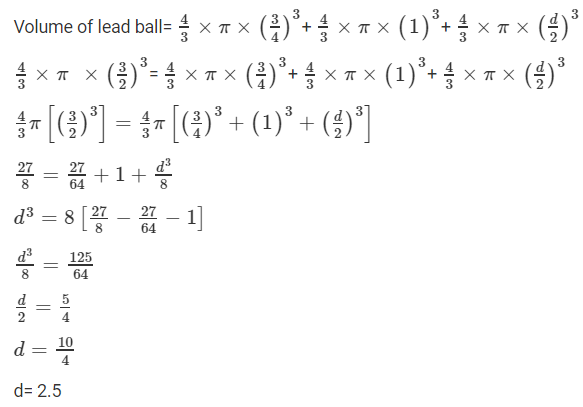So, diameter of third ball is 2.5 cm.

Question 8: A sphere of radius 5 cm is immersed in water filled in a cylinder, the level of water rises 5/3 cm. Find the radius of the cylinder.

Solution:

Radius of sphere = 5 cm (Given)

Let ‘r’ be the radius of cylinder.

We know, Volume of sphere = 4/3πr3

By putting values, we get

= 4/3×π×(5)3

Height (h) of water rises is 5/3 cm (Given)

Volume of water rises in cylinder = πr2h

Therefore, Volume of water rises in cylinder = Volume of sphere

So, πr2h = 4/3πr3

π r2 × 5/3 = 4/3 × π × (5)3

or r2 = 100

or r = 10

Therefore, radius of the cylinder is 10 cm.

Question 9: If the radius of a sphere is doubled, what is the ratio of the volume of the first sphere to that of the second sphere?

Solution:

Let r be the radius of the first sphere then 2r be the radius of the second sphere.

Now,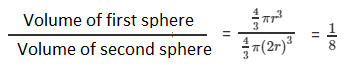Ratio of volume of the first sphere to the second sphere is 1:8.

Question 10: A cone and a hemisphere have equal bases and equal volumes. Find the ratio of their heights.

Solution:

Volume of the cone = Volume of the hemisphere (Given)

1/3πr2h = 2/3 πr3

(Using respective formulas)

r2h = 2r3

or h = 2r

Since, cone and a hemisphere have equal bases which implies they have the same radius.

h/r = 2

or h : r = 2 : 1

Therefore, Ratio of their heights is 2:1

Question 11: A vessel in the form of a hemispherical bowl is full of water. Its contents are emptied in a right circular cylinder. The internal radii of the bowl and the cylinder are 3.5 cm and 7 cm respectively. Find the height to which the water will rise in the cylinder.

Solution:

Volume of water in the hemispherical bowl = Volume of water in the cylinder … (Given)

Inner radius of the bowl ( r1) = 3.5cm

Inner radius of cylinder (r2) = 7cm

Volume of water in the hemispherical bowl = Volume of water in the cylinder

2/3πr13 = πr22h

[Using respective formulas]

Where h be the height to which water rises in the cylinder.

2/3π(3.5)3 = π(7)2h

or h = 7/12

Therefore, 7/12 cm be the height to which water rises in the cylinder.

Question 12: A cylinder whose height is two thirds of its diameter, has the same volume as a sphere of radius 4 cm. Calculate the radius of the base of the cylinder.

Solution:

Radius of a sphere (R)= 4 cm (Given)

Height of the cylinder = 2/3 diameter (given)

Let h be the height and r be the base radius of a cylinder, then

h = 2/3× (2r) = 4r/3

Volume of the cylinder = Volume of the sphere

πr2h = 4/3πR3

π × r2 × (4r/3) = 4/3 π (4)3

(r)3 = (4)3

or r = 4

Therefore, radius of the base of the cylinder is 4 cm.

Question 13: A vessel in the form of a hemispherical bowl is full of water. The contents are emptied into a cylinder. The internal radii of the bowl and cylinder are respectively 6 cm and 4 cm. Find the height of water in the cylinder.

Solution:

Radius of a bowl (R)= 6 cm (Given)

Radius of a cylinder (r) = 4 cm (given)

Let h be the height of a cylinder.

Now,

Volume of water in hemispherical bowl = Volume of cylinder

2/3 π R3 = πr2 h

2/3 π (6)3 = π(4)2 h

or h = 9

Therefore, height of water in the cylinder 9 cm.

Question 14: A cylindrical tub of radius 16 cm contains water to a depth of 30 cm. A spherical iron ball is dropped into the tub and thus level of water is raised by 9 cm. What is the radius of the ball?

Solution:

Let r be the radius of the iron ball.

Radius of the cylinder (R) = 16 cm (Given)

A spherical iron ball is dropped into the cylinder and thus the level of water is raised by 9 cm. So, height (h) = 9 cm

From statement,

Volume of iron ball = Volume of water raised in the hub

4/3πr3 = πR2h

4/3 r3 = (16)2 × 9

or r3 = 1728

or r = 12

Therefore, radius of the ball = 12cm.

### Exercise VSAQs Page No: 21.25

Question 1: Find the surface area of a sphere of radius 14 cm.

Solution:

Radius of a sphere (r) = 14 cm

Surface area of a sphere = 4πr2

= 4 × (22/7) × 142 cm2

= 2464 cm2

Question 2: Find the total surface area of a hemisphere of radius 10 cm.

Solution:

Radius of a hemisphere (r) = 10 cm

Total surface area of a hemisphere = 3πr2

= 3 × (22/7) × 102 cm2

= 942 cm2

Question 3: Find the radius of a sphere whose surface area is 154 cm2.

Solution:

Surface area of a sphere = 154 cm2

We know, Surface area of a sphere = 4πr2

So, 4πr2 = 154

4 x 22/7 x r2 = 154

r2 = 49/4

or r = 7/2 = 3.5

Radius of a sphere is 3.5 cm.

Question 4: The hollow sphere, in which the circus motor cyclist performs his stunts, has a diameter of 7 m. Find the area available to the motorcyclist for riding.

Solution:

Diameter of hollow sphere = 7 m

So, radius of hollow sphere = 7/2 m = 3.5 cm

Now,

Area available to the motorcyclist for riding = Surface area of a sphere = 4πr2

= 4 × (22/7) × 3.52 m2

= 154 m2

Question 5: Find the volume of a sphere whose surface area is 154 cm2.

Solution:

Surface area of a sphere = 154 cm2

We know, Surface area of a sphere = 4πr2

So, 4πr2 = 154

4 x 22/7 x r2 = 154

or r2 = 49/4

or r = 7/2 = 3.5

Now,

Volume of sphere = 4/3 π r3

= (4/3) π × 3.53

= 179.66

Therefore, Volume of sphere is 179.66 cm3.

### RD Sharma Solutions for Class 9 Maths Chapter 21 Surface Area and Volume of A Sphere

In the 21st Chapter of Class 9 RD Sharma Solutions students will study important concepts listed below:

• Sphere Introduction
• Section of a sphere by a plane
• Surface Area of a sphere, hemisphere and spherical shell
• Volume of a sphere, hemisphere and spherical shell

## Frequently Asked Questions on RD Sharma Solutions for Class 9 Maths Chapter 21

### What are the key features of RD Sharma Solutions for Class 9 Maths Chapter 21?

RD Sharma Solutions for Class 9 Maths Chapter 21 provides solutions to all the exercise questions accurately based on student’s intelligence quotient. The solutions offer various methods of solving tricky questions effortlessly and clear doubts immediately which arise while solving. Solving these questions enables students to analyze their preparation level and work upon the areas which need more practice.

### How RD Sharma Solutions for Class 9 Maths Chapter 21 helpful from an exam perspective?

RD Sharma Solutions for Class 9 Maths Chapter 21 is the most popular study material used by students to prepare for the board exams. Practising these solutions in a diligent manner helps the students to top the final exams and ace a subject. These solutions are devised, based on the most updated RD Sharma Solutions for Class 9 Maths Syllabus, covering all the crucial topics of the respective subject.

### What is the meaning of the volume of Sphere according to RD Sharma Solutions for Class 9 Maths Chapter 21?

According to RD Sharma Solutions for Class 9 Maths Chapter 21 the volume of a sphere is the capacity it has. The shape of the sphere is round and three-dimensional. It has three axes namely, x-axis, y-axis and z-axis which define its shape. All the things like football and basketball are examples of the sphere which have volume. The volume here depends on the diameter or radius of the sphere since if we take the cross-section of the sphere, it is a circle. The surface area of a sphere is the area or region of its outer surface.